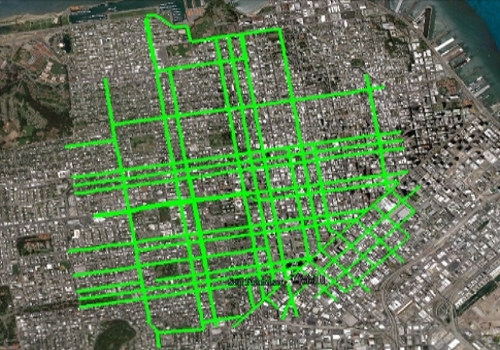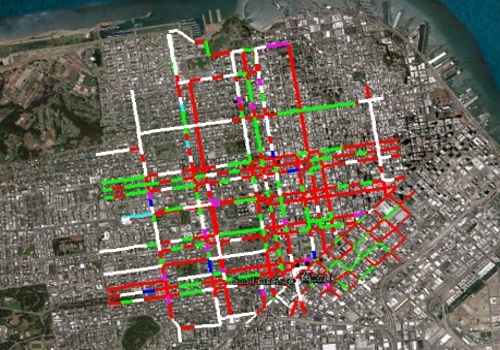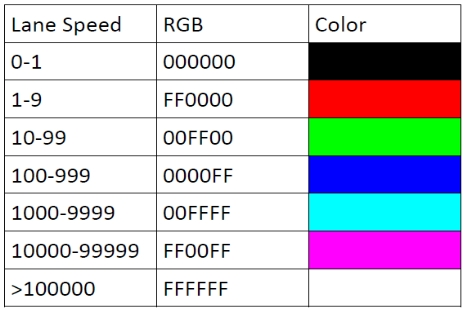# Result

## a. Average Travel Time Estimation

 Averege Lane Speed Optimized Lane SpeedWe use regularized least squares with cvx to numerically implement this optimization problem. To our surprise, the speed of lanes varies greatly, from 0 to almost infinity.

## b. Traffic Pattern Study

Our primary task in data analysis was to identify an appropriate training set from which to determine the parameters of our model. We focused on two schemes for choosing a validation set, which had surprising results given our other analyses.

The first and simpler of the schemes we studied was to simply choose some portion of the earliest observations – Our first attempt used the first 3000 of the approximately 4500 measurements as the training set, and the remainder as the validation set. This approach yielded reasonable results for the time necessary to construct the training set.
The second method we tried was randomly selecting a percentage of the observations for use as the validation set. While less easy to implement, this converged to an accurate model faster (using a smaller proportion of the observations) than the naïve block approach we used first.

A surprising behavior of both of these methods is that they did not appear to lose accuracy as the size of the validation set in proportion to the training set increased; i.e. neither method produced ‘overtrained’ models. As is clearly visible in figures 3 and 4 of the attachment, both schemes exhibit a linear relationship between size of the validation set and model accuracy, with optimal error being achieved with training sets comprised of 90% of the data. This behavior is surprising given the apparent independence of the observed data. Singular value decomposition of the matrix of observations showed that the first 700 singular values (of a total of 817) differed only by a factor of order unity, suggesting that the routes taken by the probes was highly uncorrelated.

Perhaps owing to their similarity, the different K matrices we experimented with seemed to have no distinct effects on the model produced, yielding very similar results given training sets and optimization parameters.